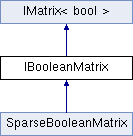MyMediaLite  3.11
IBooleanMatrix Interface Reference

Interface for boolean matrices More...

Inheritance diagram for IBooleanMatrix:## Public Member Functions

IMatrix< T > CreateMatrix (int num_rows, int num_columns)
Create a matrix with a given number of rows and columns More...

IList< int > GetEntriesByColumn (int column_id)
Get all true entries (row IDs) of a column More...

IList< int > GetEntriesByRow (int row_id)
Get all true entries (column IDs) of a row More...

int NumEntriesByColumn (int column_id)
Get all the number of entries in a column More...

int NumEntriesByRow (int row_id)
Get all the number of entries in a row More...

int Overlap (IBooleanMatrix s)
Get the overlap of two matrices, i.e. the number of true entries where they agree More...

void Resize (int num_rows, int num_cols)
Grows or shrinks the matrix to the requested size, if necessary More...

IMatrix< T > Transpose ()
Get the transpose of the matrix, i.e. a matrix where rows and columns are interchanged More...

## Properties

bool IsSymmetric` [get]`
True if the matrix is stored in a symmetric manner, false otherwise More...

IList< int > NonEmptyColumnIDs` [get]`
The IDs of the non-empty columns in the matrix (the ones that contain at least one true entry) More...

IList< int > NonEmptyRowIDs` [get]`
The IDs of the non-empty rows in the matrix (the ones that contain at least one true entry) More...

int NumberOfColumns` [get]`
The number of columns of the matrix More...

int NumberOfEntries` [get]`
The number of (true) entries More...

int NumberOfRows` [get]`
The number of rows of the matrix More...

this[int x, int y]` [get, set]`
The value at (i,j) More...

ICollection< int > this[int x]` [get]`
Indexer to access the rows of the matrix More...

## Detailed Description

Interface for boolean matrices

## Member Function Documentation

 IMatrix CreateMatrix ( int num_rows, int num_columns )
inherited

Create a matrix with a given number of rows and columns

Parameters
 num_rows the number of rows num_columns the number of columns
Returns
A matrix with num_rows rows and num_column columns
 IList GetEntriesByColumn ( int column_id )

Get all true entries (row IDs) of a column

Parameters
 column_id the column ID
Returns
a list of row IDs

Implemented in SparseBooleanMatrix.

 IList GetEntriesByRow ( int row_id )

Get all true entries (column IDs) of a row

Parameters
 row_id the row ID
Returns
a list of column IDs

Implemented in SparseBooleanMatrix.

 int NumEntriesByColumn ( int column_id )

Get all the number of entries in a column

Parameters
 column_id the column ID
Returns
the number of entries in column column_id

Implemented in SparseBooleanMatrix.

 int NumEntriesByRow ( int row_id )

Get all the number of entries in a row

Parameters
 row_id the row ID
Returns
the number of entries in row row_id

Implemented in SparseBooleanMatrix.

 int Overlap ( IBooleanMatrix s )

Get the overlap of two matrices, i.e. the number of true entries where they agree

Parameters
 s the IBooleanMatrix to compare to
Returns
the number of entries that are true in both matrices

Implemented in SparseBooleanMatrix.

 void Resize ( int num_rows, int num_cols )
inherited

Grows or shrinks the matrix to the requested size, if necessary

The new entries are filled with zeros. Obsolete entries are removed.

Parameters
 num_rows the number of rows num_cols the number of columns
 IMatrix Transpose ( )
inherited

Get the transpose of the matrix, i.e. a matrix where rows and columns are interchanged

Returns
the transpose of the matrix (copy)

## Property Documentation

 bool IsSymmetric
getinherited

True if the matrix is stored in a symmetric manner, false otherwise

Also true for skew symmetric matrices that exploit this property in order to save memory

 IList NonEmptyColumnIDs
get

The IDs of the non-empty columns in the matrix (the ones that contain at least one true entry)

 IList NonEmptyRowIDs
get

The IDs of the non-empty rows in the matrix (the ones that contain at least one true entry)

 int NumberOfColumns
getinherited

The number of columns of the matrix

The number of columns of the matrix

 int NumberOfEntries
get

The number of (true) entries

 int NumberOfRows
getinherited

The number of rows of the matrix

The number of rows of the matrix

 T this[int x, int y]
getsetinherited

The value at (i,j)

The value at (i,j)

Parameters
 x the row ID y the column ID
 ICollection this[int x]
get

Indexer to access the rows of the matrix

Parameters
 x the row ID

The documentation for this interface was generated from the following file:
• IBooleanMatrix.cs